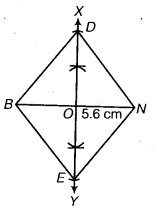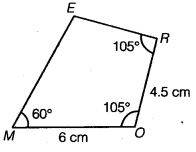# Class 8 Maths NCERT Solutions for Chapter – 4 Practical Geometry Ex – 4.2

## Practical Geometry

Question 1.

LI = 4 cm
IF = 3 cm
TL = 2.5 cm
LF = 4.5 cm
IT = 4 cm

OL = 7.5 cm
GL = 6 cm
GD = 6 cm
LD = 5 cm
OD = 10 cm

(iii) Rhombus BEND
BN – 5.6 cm
DE = 6.5 cm

Solution.
(i) Let us draw a rough sketch of the required quadrilateral and write down the dimensions. Clearly, the two easily constructible triangles are UT and LIF.

Steps of Construction :• Draw LI = 4 cm.
• With L as centre and radius 2.5 cm, draw an arc.
• With I as centre and radius 4 cm draw another arc to cut the previous arc at T.
• Join TL and 77.
• With L as centre and radius 4.5 cm, draw an arc.
• With I as centre and radius 3 cm, draw another arc to cut the previously drawn arc at F.
• Join FI, FL and TF.
Then, LIFT is the required quadrilateral.

(ii) Let us draw a rough sketch of the required quadrilateral and write down its dimensions. Clearly, the two easily constructible triangles are LOD and LGD.

Steps of Construction :• Draw LD = 5 cm.
• With L as centre and radius 7.5 cm, draw an arc.
• With D as centre and radius 10 cm, draw another arc to cut the previous arc at O.
• Join OL and OD.
• With L as centre and radius 6 cm, draw an arc.
• With D as centre and radius 6 cm, draw an arc to cut the previously drawn arc at B.
• Join GD, GL and OG.
Then, GOLD is the required quadrilateral.

(iii) We know that the diagonals of a rhombus bisect each other at right angles. So, we proceed according to the following steps.

Steps of Construction :• Draw BN = 5.6 cm.
• Draw the right bisector XY of BN, meeting BN at O.
• From O set off OE =$\frac { 1 }{ 2 }$ (6.5) cm = 3.25 cm along OY and OD = 3.25 cm along OX.
• Join BE, EN, ND and DB.
Then, BEND is the required rhombus.

### Take Your IIT JEE Coaching to Next Level with Examtube

• Mentoring & Teaching by IITians
• Regular Testing & Analysis
• Preparation for Various Engineering Entrance Exams
• Support for School/Board Exams